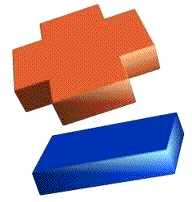# Addition and Subtraction English lesson learning the basics

## What will I be learning from the lesson learning the basics in addition and subtraction?

This lesson you will learn how to do some basic maths addition and subtraction you will learn the vocabulary used and how to add and subtract.

** Hint ** You know how to do maths when it is on your own language, but it been able to do it  English it will be very useful to you.

Easy Pace Learning has a lesson Learning English cardinal - numbers 1 to 100 in English if you need to refresh your knowledge on numbers

### When using addition the following English vocabulary is used.+ plus + and = equals

3
+
7
=
10
three
plus
seven
equals
ten
9
+
6
=
15
nine
plus
six
is
fifteen

What's three (3)  plus four (4)? = seven (7)

What's two (2)  plus five (5)? = seven (7)

### When using subtraction the following English vocabulary is used.

 - minus - take away = equals

### Subtraction or Subtracting

7      -    3    =   4
seven           minus three            equals         four
9     -    6    =   3
nine take away                 six    is three

What's five (5)  plus two (2)? = three (3)

What's nine (9)  plus six (6)? = three (73)

### Some different examples of addition and subtraction a person or teacher might say.

What would you get if you added three and five together?
You would get eight.

If you put six and three together, what would you get?
You would get nine.

If you add 10 to 25, what does that make?
That makes 35.

What do you get if you subtract five from nine?
You get four.

How much do you have if you take nine away from seven?
You have two.

### Practise with some paper and a pen Using the previous examples make up your own questions and answer them.

Use words for the numbers for extra practise!

what is four (4) plus four(4) ?    it is eight (8)

what is Seven minus two ? it is five

### Lessons that are related to this one

To view a lesson just click on the link.

English Numbers 1 to 100 learning cardinal numbers

Learning English numbers video learning English

## Easy pace Learning online dictionary and how to use dictionaries

Click on the following link for the Online English dictionary - English lesson